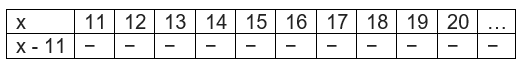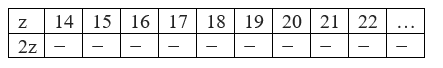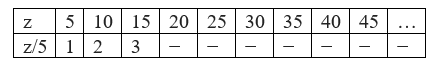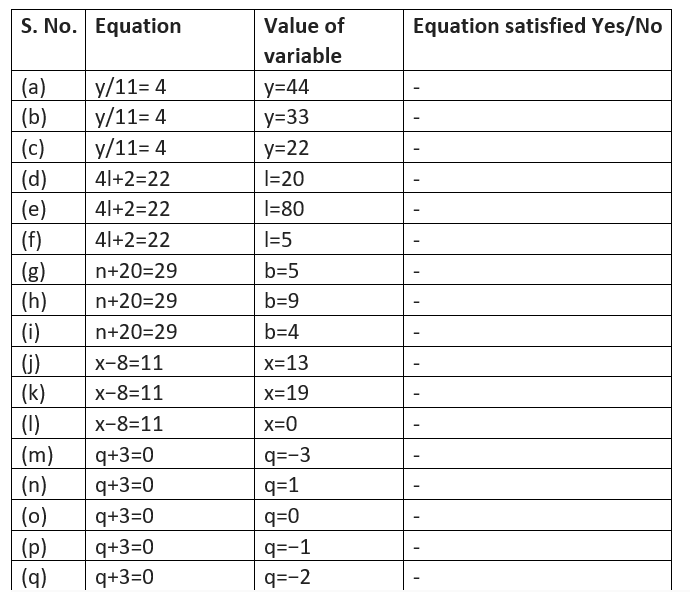# Important Questions for Class 6 Maths algebra

In this page we have Important Questions for Class 6 Maths algebra . Hope you like them and do not forget to like , social share and comment at the end of the page.
Topics of Use of variables in common rules,Expressions with variables,Using expressions practically,What is an equation?,Solution of an equation is deleted from this chapter as per rationalized syllabus. So you may ignore those questions

Question 1 Give expressions for the following cases.
(b) 99 subtracted from m
(c) n multiplied by 8
(d) p divided by 10
(e) 11 subtracted from − x (f) − y multiplied by 1
(g) − x divided by 11
(h) z multiplied by – 5
Question 2
Which out of the following are expressions with both variable and numbers only?
(a) $p -9$
(b) $(11 \times 20) - 8x$
(c) $5 (21 - 7) + 7 \times 2+p$
(d) $1$
(e) $11x-1$ (f) $5 +4+3+2+1$
(g) $(7 \times 8) - (8 \times 7) - 45 +11x$
(i) $2z-11$
Question 3
Get the algebraic expressions in the following cases using variables, constants and arithmetic operations.
(i) Subtraction of z from y.
(ii) One-half of the sum of numbers a and b.
(iii) The number y multiplied by itself.
(iv) One-Eighted of the product of numbers x and y.
(v) Numbers p , q and r  both squared and added.
(vi) Number 5 added to three times the product of number m and n.
(vii) Product of numbers y and z subtracted from 10.
(viii)Sum of numbers x and y subtracted from their product.
Question 4
(a) Complete the table and by inspection of the table, find the solution to the equation x -11 = 6(b) Complete the table and by inspection of the table, find the solution to the equation 2z = 44(c) Complete the table and find the solution of the equation z/5 = 6 using the table.Question 5
Complete the entries in the third column of the table.### Practice Question

Question 1 What is $\frac {1}{2} + \frac {3}{4}$ ?
A)$\frac {5}{4}$
B)$\frac {1}{4}$
C)$1$
D)$\frac {4}{5}$
Question 2 Pinhole camera produces an ?
A)An erect and small image
B)an Inverted and small image
C)An inverted and enlarged image
D)None of the above